Next: 3.3 Approximations to Geometric Up: 3.2 Solving the Level Previous: 3.2.3 Stability

## 3.2.4 Surface Velocity Extension

In topography simulations the velocity field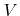has no physical meaning, since the surface velocity can only be determined at points on the surface, where the surface velocity corresponds to the local deposition or etch rate. The LS method, however, requires a velocity field, for which the values must be known for at least all grid points which are updated in time. Hence, to enable the solution of the LS equation a technique is required which extrapolates the surface velocities from the surface to all those grid points.

Assuming that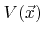is available for all points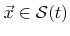, different techniques have been developed to obtain a surface velocity field which allows a stable time integration of the LS equation. In , it was proposed to define the surface velocity field as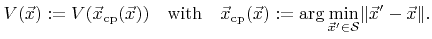(3.17)

Hence, the surface velocity at a certain point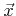is set to the velocity of its closest surface point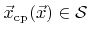. Since the velocity field needs to be known for all grid points, all their corresponding closest surface points must be determined.

A less costly technique to extrapolate the surface velocities based on an alternative formulation of the problem  is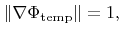(3.18)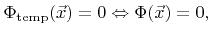(3.19)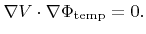(3.20)

Here, the first two equations define a signed distance function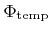with the same zero LS as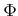. The solution of this boundary value problem fulfills (3.17). The system of equations can be efficiently solved using the fast marching method . With this finite difference scheme the surface velocity field can be obtained for all grid points with an optimal linear complexity.Next: 3.3 Approximations to Geometric Up: 3.2 Solving the Level Previous: 3.2.3 Stability

Otmar Ertl: Numerical Methods for Topography Simulation